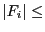Next: Solving univariate polynomial with Up: Krawczyk method for solving Previous: Mathematical background   Contents

Implementation

The procedure is implemented as:

int Krawczyk_Solver(int m,int n,
INTERVAL_VECTOR (* IntervalFunction)(int,int,INTERVAL_VECTOR &),
INTERVAL_MATRIX (* IntervalGradient)(int, int, INTERVAL_VECTOR &),
INTERVAL_VECTOR &Input,double Acc , VECTOR &Result)
with
• m: the number of unknown
• n: the number of equations
• IntervalFunction: a function which return the interval vector evaluation of the equations, see the note 2.3.4.3
• IntervalGradient: a function which the interval matrix of the jacobian of the equations, see the note 2.4.2.2
• Input: the ranges for the variables
• Acc: the algorithm will return the result ifAcc
• Result: the solution of the system
The procedure will return 1 if it has converged to a solution, 0 or -1 otherwise.

Jean-Pierre Merlet 2012-12-20